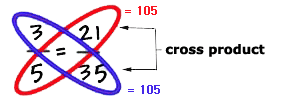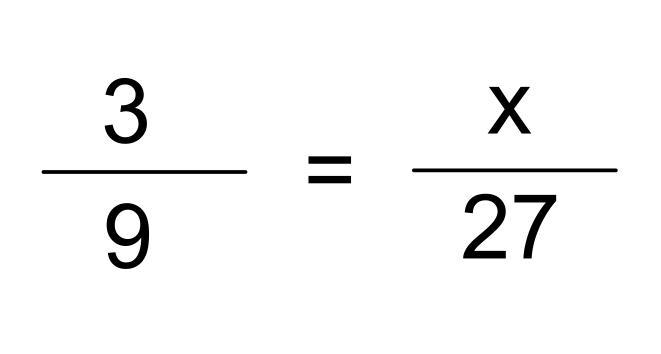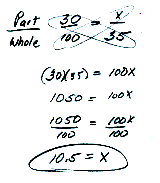• RATIO is two "things" (numbers or quantities) compared to each other. or quantities.

For example, "3 dollars per gallon" is a ratio.
Or, "40 miles per 1 hour".
Or, 15 girls versus 14 boys.
Or, 569 words in 2 minutes.
Or, 23 green balls to 41 blue balls, etc.

PROPORTION is when you have two ratios set to be equal to each other.

For example, 3 dollars per gallon equals 6 dollars per two gallons. Or,  3/1 = 6/2
2 teachers per 20 kids equals 3 teachers per 30 kids.  Or, 2/20 = 3/30

Hey! That look's like a pair of equivalent fractions.  Right, fractions are rational numbers.  When two fractions represent the same amount, they are equivalent fractions.  Two ratios that are equivalent to each other are a proportion.

How do you know if two ratios are a proportion?

• An equation such as 5/8 = 60/96 is a proportion if the two ratios are equivalent to each other.  Either reduce one ratio to lowest terms or raise the other ratio to equivalent terms.

5 x 12 = 60         8 x 12 = 96     therefore 5/8 = 60/96

60 / 12 = 5          96 / 12 = 8     therefore 5/8 = 60/96

• Another way to test a proportion for is to use cross multiplication.Multiply 3 by 35.   3 x 35 = 105

Multiply 21 by 5.    21 x 5 = 105

Both products are 105, therefore the ratios are proportional.

Of course for it to be a PROBLEM, one of the four numbers is missing or ends up being the famous x.

What if one term in a proportion is missing?  How can you find the missing term?
• One way to find a missing term in a proportion is to find the equivalent fraction.27 is a multiple of 9 by 3, therefore do the same to the numerator.  3 times 3 equals 9, therefore x is 9.
• A second way to find a missing term in a proportion is to use cross multiplication.Real-life Applications
• Maps, blueprints, and scale models.  An appropriate ratio, or scale, is chosen.  Large objects or areas can then be shown in a considerably smaller size or space. Movies  frequently use scale models of ship, planes, buildings.
• Recipes or formulas may be increased or decreased by using proportions.

• Economic and sociological predictions based on the relationship of two quantities.